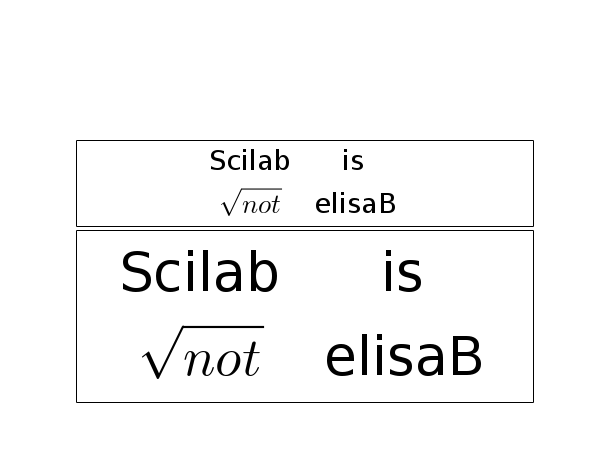Scilab Home page | Wiki | Bug tracker | Forge | Mailing list archives | ATOMS | File exchange
Change language to: Français - Português - 日本語 - Русский

See the recommended documentation of this function

Scilab Help >> Graphics > text > xstringb

# xstringb

draw strings into a box

### Syntax

xstringb(x,y,str,w,h,[option])

### Arguments

x,y,w,h

vector of 4 real scalars defining the box.

str

matrix of strings.

Starting from Scilab 5.2, it is possible to write LaTeX or MathML expression.

option

string.

### Description

xstringb draws the matrix of strings str centered inside the rectangle rect=[x,y,w,h] (lower-left point, width, height) in user coordinates.

If option is given with the value "fill", the character size is computed so as to fill as much as possible in the rectangle.

Enter the command xstringb() to see a demo.

### Examples

str=["Scilab" "is";"$\sqrt{not}$" "elisaB"];
plot2d(0,0,[-1,1],"010"," ",[0,0,1,1]);

r=[0,0,1,0.5];
xstringb(r(1),r(2),str,r(3),r(4),"fill");
xrect(r(1),r(2)+r(4),r(3),r(4));

r=[r(1),r(2)+r(4)+0.01,r(3),r(4)/2];
xrect(r(1),r(2)+r(4),r(3),r(4))
xstringb(r(1),r(2),str,r(3),r(4),"fill");• titlepage — add a title in the middle of a graphics window
• xstring — draw strings
• xstringl — compute a box which surrounds strings
• xtitle — add titles on a graphics window